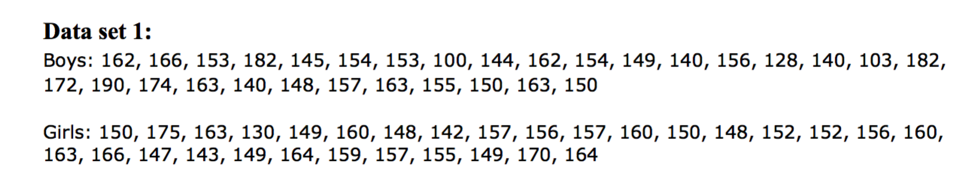# Descriptive Statistics

## Objective

Develop and answer statistical questions through data analysis of existing data using appropriate statistical measures and displays. (Part 1/3)

## Common Core Standards

### Core Standards

?

• HSS-ID.A.1 — Represent data with plots on the real number line (dot plots, histograms, and box plots).

• HSS-ID.A.2 — Use statistics appropriate to the shape of the data distribution to compare center (median, mean) and spread (interquartile range, standard deviation) of two or more different data sets.

• HSS-ID.A.3 — Interpret differences in shape, center, and spread in the context of the data sets, accounting for possible effects of extreme data points (outliers).

• HSS-ID.A.4 — Use the mean and standard deviation of a data set to fit it to a normal distribution and to estimate population percentages. Recognize that there are data sets for which such a procedure is not appropriate. Use calculators, spreadsheets, and tables to estimate areas under the normal curve.

?

• 7.SP.A.1

• 7.SP.A.2

• 7.SP.B.3

• 7.SP.B.4

## Criteria for Success

?

1. Develop two statistical questions—one that requires categorical data and one that requires numerical data.
2. Define the variable you are measuring and relevant units, and explain why the variable represents either quantitative or categorical data.
3. Identify visual models you will use to illustrate the variables chosen. Identify the benefits and drawbacks of each visual model.

## Tips for Teachers

?

• This is the first of three project-based lessons on univariate data. There is a second set of three project-based lessons on bivariate data at the end of this unit.
• There is one basic context that is included in this series of three lessons, but the data sets can be swapped out easily using the resources in the problem set guidance or to meet the interests or needs of your students.
• Experimentation and data collection is encouraged, although there are a lot of readily available data sets to use as well. Additional data sets and options are included in the problem set guidance.
• The target task for this lesson is a reflection of the work done in class and is not meant to be an assessment of how well students have completed this part of the project.
• The teacher will need to develop an appropriate rubric to emphasize the portions of the modeling cycle that are most relevant to their students’ development in this process. See the SIAM modeling guide, Math Modeling: Getting Started and Getting Solutions, for more information.

## Anchor Problems

?

Below is a data set of the length of the arm span of a group of boys and another group of girls:What statistical question could you answer using these data sets?

#### References

STatistics Education Web (STEW) Grades 6-8, Armspans

## Problem Set

?

The following resources include problems and activities aligned to the objective of the lesson that can be used to create your own problem set.